# Exponential Function

(redirected from Exp(x))
Also found in: Dictionary, Thesaurus.
Related to Exp(x): exponential function

## exponential function

[‚ek·spə′nen·chəl ′fəŋk·shən]
(mathematics)
The function ƒ(x) = e x , written ƒ(x) = exp (x).
McGraw-Hill Dictionary of Scientific & Technical Terms, 6E, Copyright © 2003 by The McGraw-Hill Companies, Inc.
The following article is from The Great Soviet Encyclopedia (1979). It might be outdated or ideologically biased.

## Exponential Function

the important elementary function f(z) = ez; sometimes written exp z. It is encountered in numerous applications of mathematics to the natural sciences and engineering. For any real or complex value of z, the exponential function is defined by the equation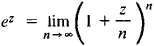It is obvious that e0 = 1. When z = 1, the value of the function is equal to e, which is the base of the system of natural logarithms. Basic properties of the function are

ez1ez2 = ez1 + z2 (ez1)z2 = ez1z2

for any values of z1 and z2. Moreover, on the real axis (Figure 1), ex > 0. As x → ∞, the function increases faster than any power of x; when x → – ∞, it decreases faster than any power of 1/x: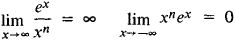no matter what the exponent n. The logarithmic function is the inverse of the exponential function: if w = ez, then z = 1n w.

The function az, where the base a > 0 is different from e, is also called an exponential function. For example, in school mathematics courses such exponential functions as 2x and (1/2)x are discussed for real values of z = x. The relation between the exponential function az and the exponential function ez is given by the equation

az = ez 1n a

The exponential function ex is an integral transcendental function. It can be expanded in the power series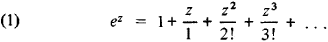which converges throughout the z-plane. Equation (1) can also serve as a definition of the exponential function.

Letting z = x + iy, L. Euler obtained (1748) the formula

(2) ez = ex + iy =ex (cos y + i sin y)

which connects the exponential function with the trigonometric functions. The equations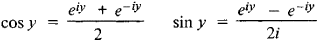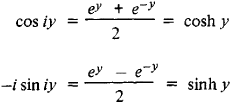are called hyperbolic functions and have a number of properties similar to those of the trigonometric functions. They and the trigonometric functions play an important role in various applications of mathematics.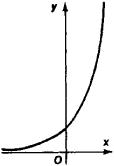Figure 1

It follows from equation (2) that the exponential function of a complex variable z has a period 2πi; that is, ez + 2πi = ez or e2πi = 1. The derivative of the exponential function is equal to the function itself: (ez)ʹ = ez.

These properties of the exponential function account for its numerous applications. In particular, the exponential function expresses the law of natural growth, which governs the course of processes whose rate is proportional to the current value of a variable quantity. Unimolecular chemical reactions and, under certain conditions, the growth of a bacteria colony are examples. The periodicity of an exponential function of a complex variable, along with the function’s other properties, accounts for the exceptionally important role the function plays in the study of all periodic processes, particularly oscillations and wave propagation.